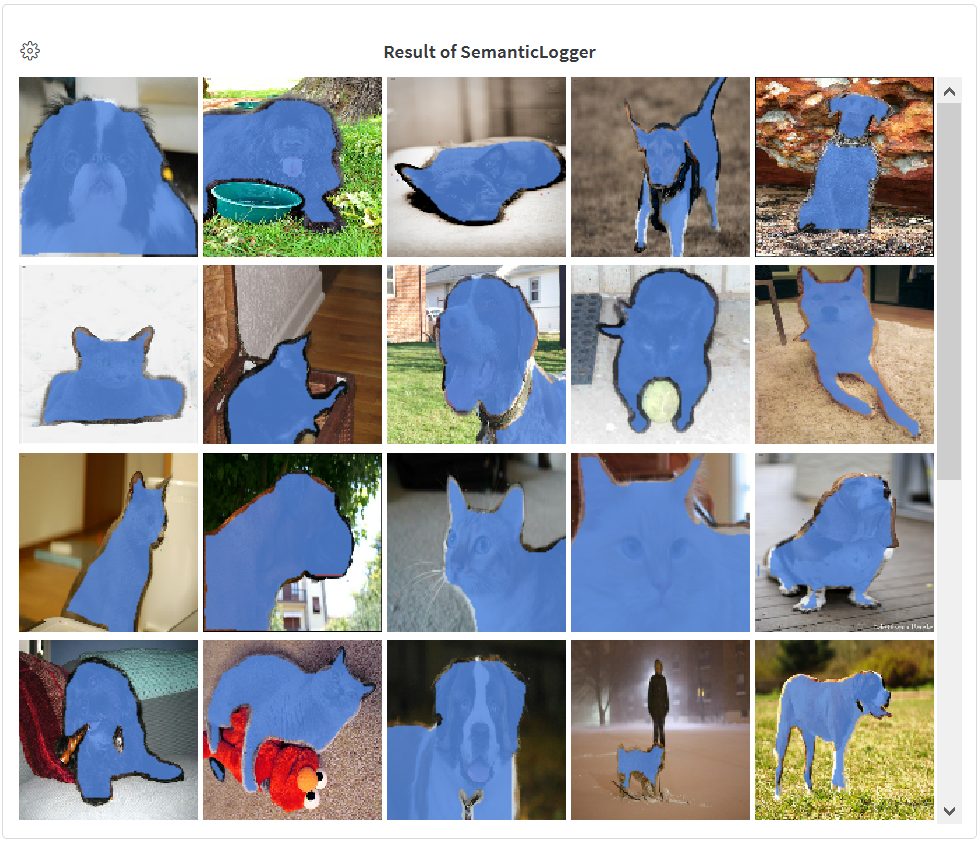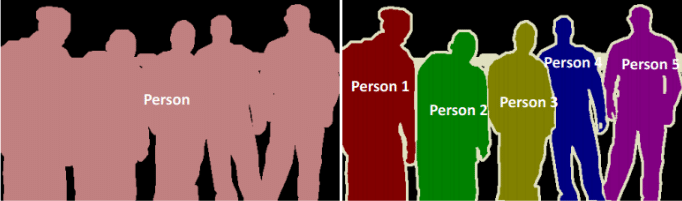# Image Segmentation using Keras and W&BAre you interested to know where an object is in the image? What is the shape of the object? Which pixels belong to the object? To accomplish this, we need to segment the image, i.e., classify each pixel of the image to the object it belongs to or give each pixel of the image a label contrary to giving one label to an image. Image Segmentation using Keras and W&B

## Introduction

Are you interested to know where an object is in the image? What is the shape of the object? Which pixels belong to the object? To accomplish this, we need to segment the image, i.e., classify each pixel of the image to the object it belongs to or give each pixel of the image a label contrary to giving one label to an image.

Thus, image segmentation is the task of learning a pixel-wise mask for each object in the image. Unlike object detection, which gives the bounding box coordinates for each object present in the image, image segmentation gives a far more granular understanding of the object(s) in the image.Figure 1: Semantic segmentation and Instance segmentation.

Image segmentation can be broadly divided into two types:

• Semantic segmentation: Here, each pixel belongs to a particular class. The left image in figure 1 is an example of semantic segmentation. The pixels either belong to the person(a class) or background(another class).
• Instance segmentation: Here, each pixel belongs to a particular class. However, pixels belonging to discrete objects are labeled with a different color(mask value). The right image in figure 1 is an example of instance, segmentation. The pixels belonging to the person’s class are colored differently.

This report will build a semantic segmentation model and train it on  Oxford-IIIT Pet Dataset. We will interactively visualize our model’s predictions in Weights & Biases.

## Keras with TensorFlow Course - Python Deep Learning and Neural Networks

Keras with TensorFlow Course - Python Deep Learning and Neural Networks for Beginners Tutorial: How to use Keras, a neural network API written in Python and integrated with TensorFlow. We will learn how to prepare and process data for artificial neural networks, build and train artificial neural networks from scratch, build and train convolutional neural networks (CNNs), implement fine-tuning and transfer learning, and more!

## Keras Tutorial - Ultimate Guide to Deep Learning - DataFlair

Keras Tutorial - Learn Keras Introduction, installation, Keras Features, Applications of Keras, Keras Layers, Keras models and keras visualize training.

## Top 10 Deep Learning Sessions To Look Forward To At DVDC 2020

Looking to attend an AI event or two this year? Below ... Here are the top 22 machine learning conferences in 2020: ... Start Date: June 10th, 2020 ... Join more than 400 other data-heads in 2020 and propel your career forward. ... They feature 30+ data science sessions crafted to bring specialists in different ...

## Why Learn Keras - Reasons Why Choose Keras - DataFlair

Learn the Features of Keras that make it worth to learn keras like backend support, the basis of innovation, and the simplicity of Keras API etc.

## OCR with Keras, TensorFlow and Deep Learning - part 1

In this tutorial, you will learn how to train an Optical Character Recognition (OCR) model using Keras, TensorFlow, and Deep Learning. This post is the first in a two-part series on OCR with Keras and TensorFlow: